# relationship 是什么

## relationship 的使用

### 模型类之间进行关联

``````class Article(db.Model):
id = db.Column(db.Integer, primary_key=True,autoincrement=True)
article_name = db.Column(db.String(20), nullable=False)
# TypeError: column() got an unexpected keyword argument 'nullable'
# 是因为article_text = db.column(db.String(500), nullable=False)  这里写了小写的c , 应该是大写的
article_text = db.Column(db.String(500), nullable=False)
create_time = db.Column(db.DateTime, default=datetime.now)
# 外键约束， 记录文章是哪个用户发表的
uid = db.Column(db.Integer, db.ForeignKey('user.id'), nullable=False)

def __str__(self):
return self.article_name

class User(db.Model):
"""
"""
id = db.Column(db.Integer, primary_key=True, autoincrement=True)
phone = db.Column(db.String(11), unique=True)
email = db.Column(db.String(20))
rdatetime = db.Column(db.DateTime, default=datetime.now)
isDelete = db.Column(db.Integer, default=0)
# 给 Article 模型插入了一个 user  属性
article = db.relationship('Article', backref='user')

def __str__(self):

### 外键约束在视图函数中进行处理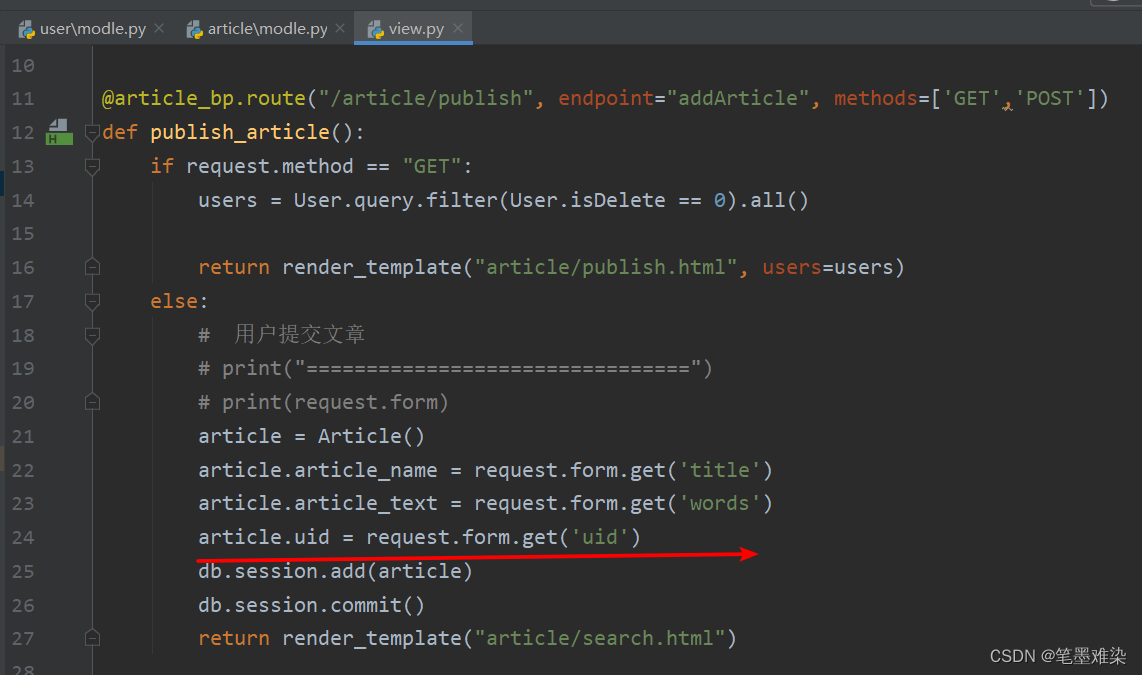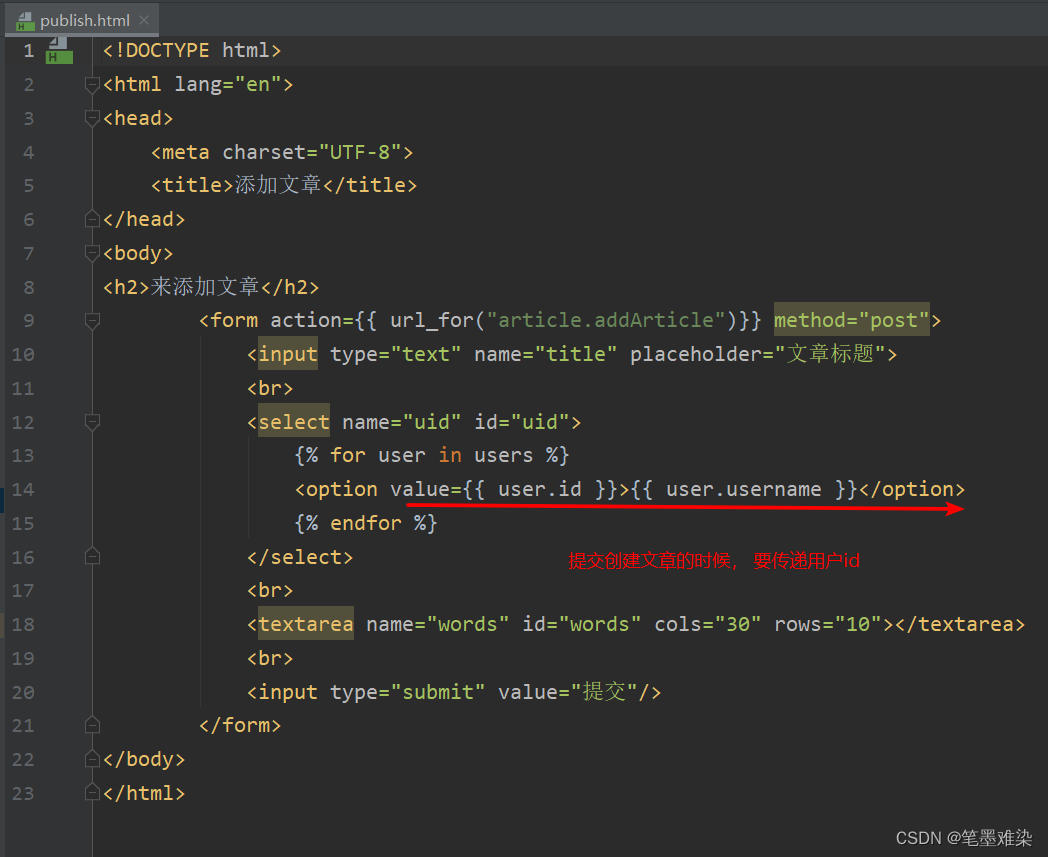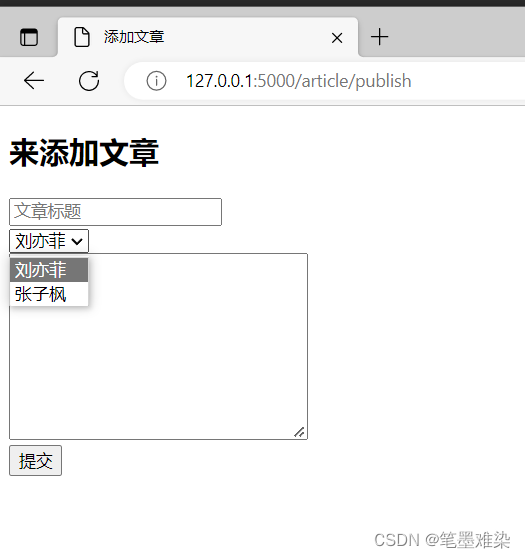### relationship的正向解析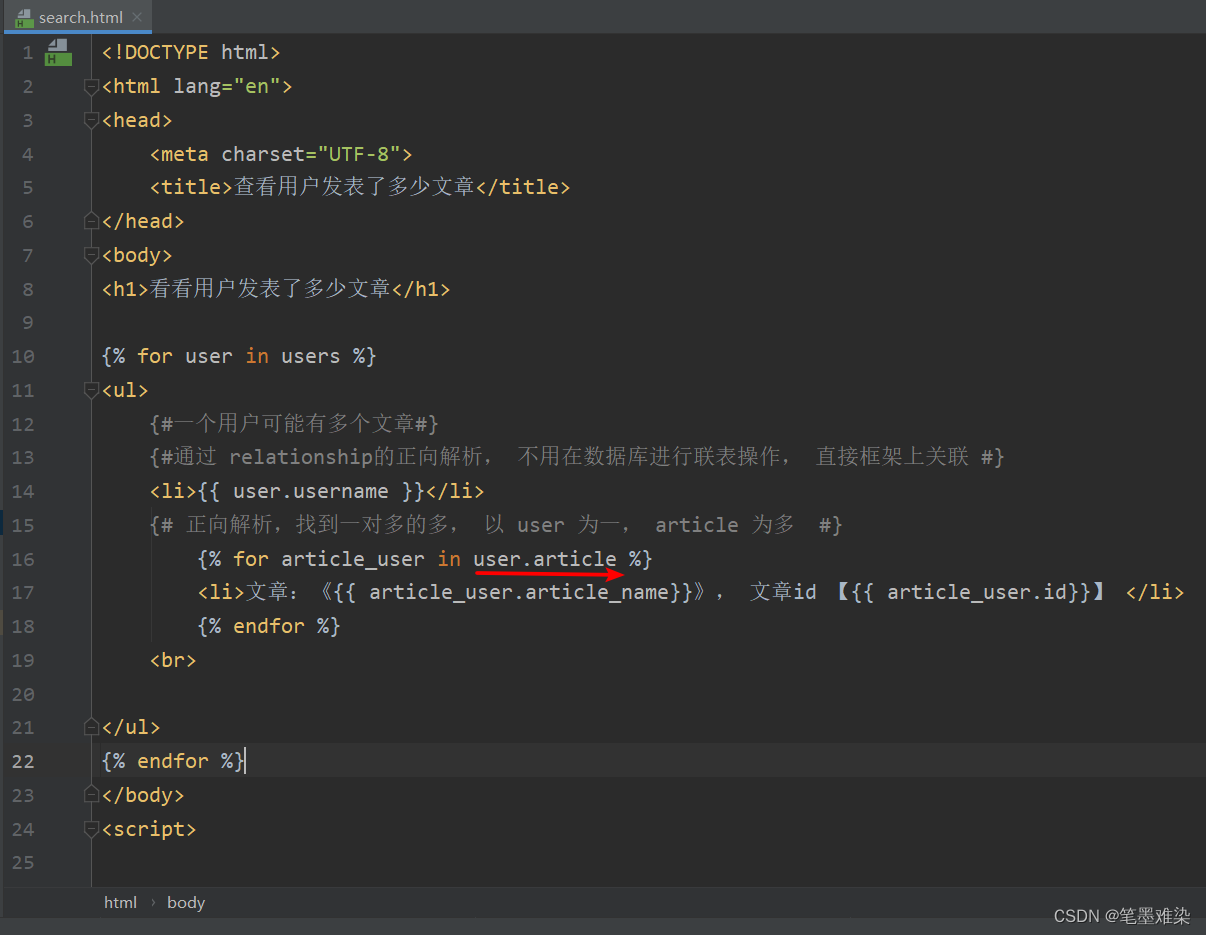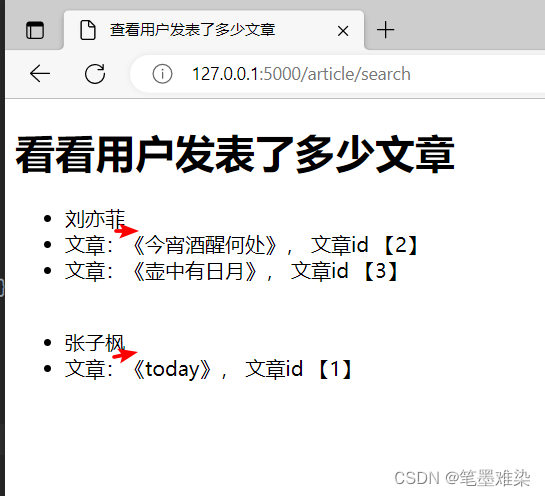### relationship的反向解析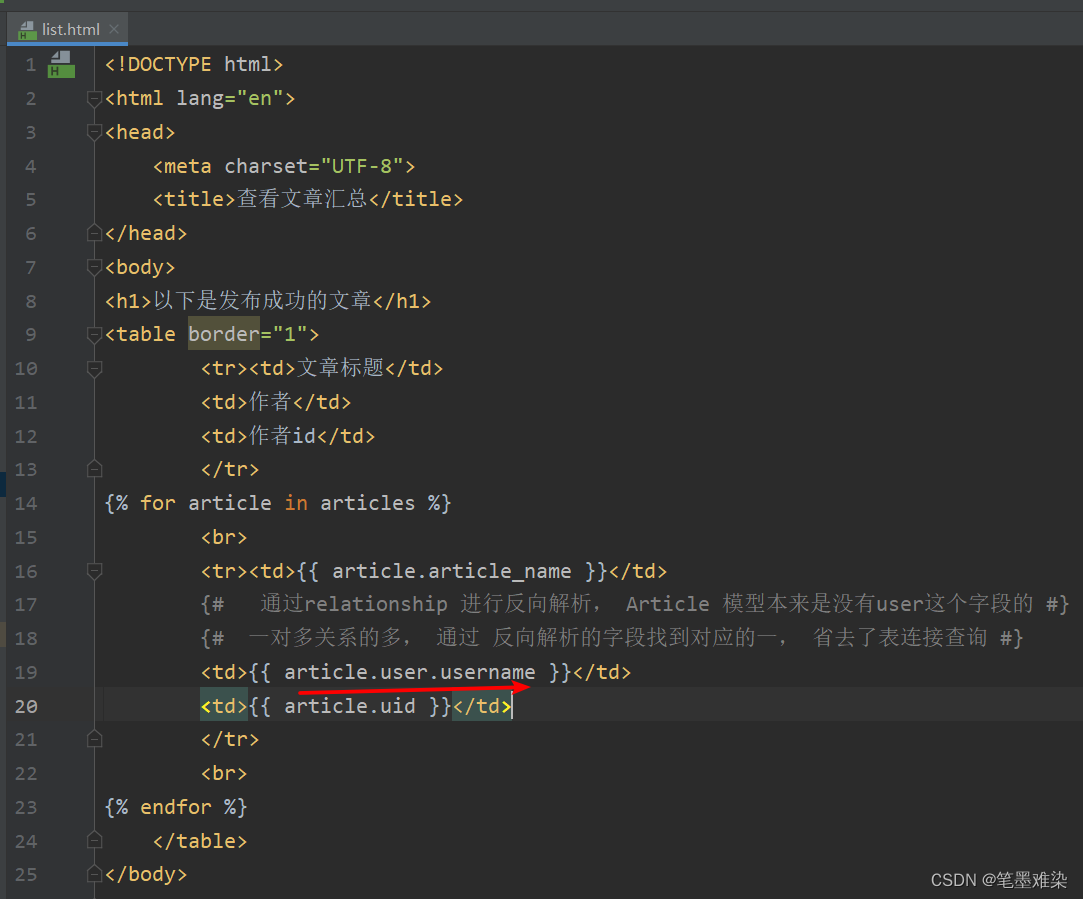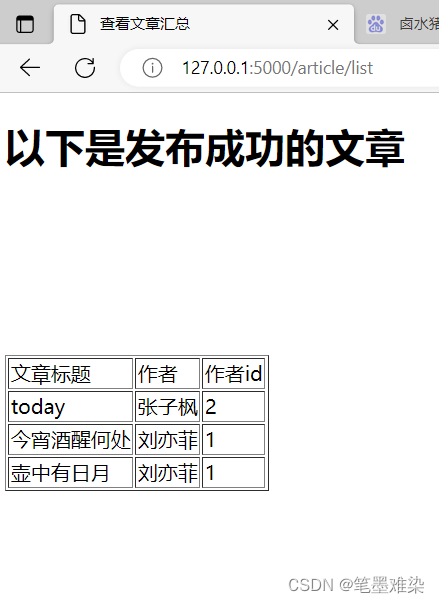### 数据库里的数据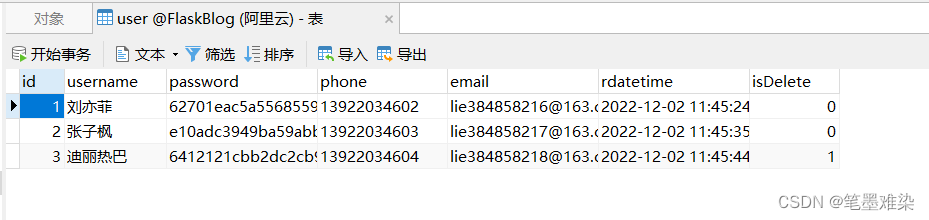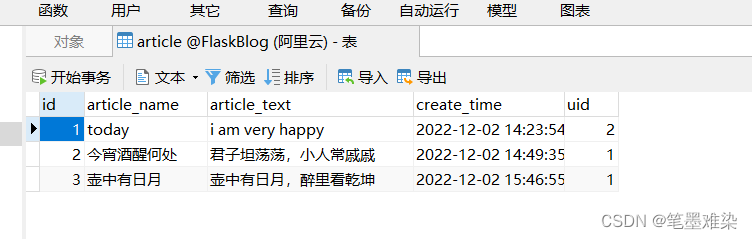## 特别注意事项

上一篇flask用到的资料网站

2022-12-05Flask踩坑之模型配置和mysql迁移同步

2022-11-17
目录